Project Management Central

HELP in this risk question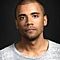Network:39Seller A may provides the materials at time with probability 60% and confidence level 55%.
Seller B may provides the materials at time with probability 40% and confidence level 90%.
You will select -----------------.
A) select B because the confidence higher than A.
B) Select A because the probability higher than B.
C) Data insufficient.
D) Select B because the selection based one cumulative value of probability and confidence level.
Sort By: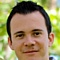Network:18I would say D (preferred) or C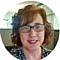Network:2980D because 55% of 60 is 33% and 90% of 40 is 36%. So I am going with the higher confidence * probability.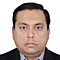Network:1160Select A because the probability higher than B.
Because in ( B ) , Probability decreases 20% sharp though high increase in confidence however if you have substantial data ( C ) to justify 90% confidence then Review Again .Network:104007This is just like your other risk question, Mohamed. Unless told otherwise, you can see that others are also assuming that probability is the likelihood of success, rather than failure. They are also applying the formula I gave you.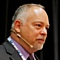Network:114883For the question D. Resolution similar to your other post.
In real life would request more informationNetwork:1633I too would select D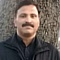Network:4562I too would select D